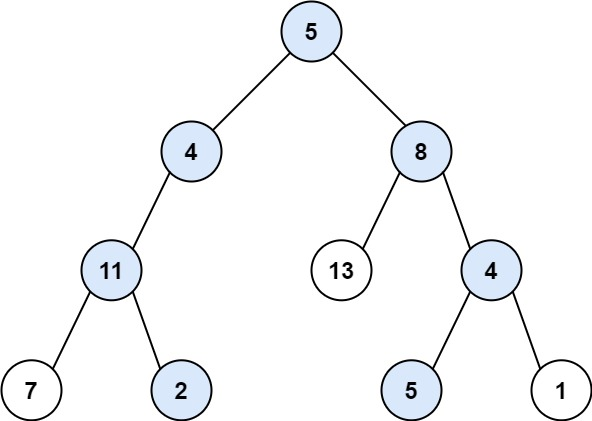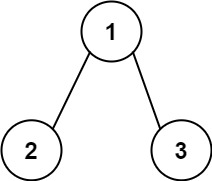# 113. Path Sum II

Medium
Given the `root` of a binary tree and an integer `targetSum`, return all root-to-leaf paths where the sum of the node values in the path equals `targetSum`. Each path should be returned as a list of the node values, not node references.
A root-to-leaf path is a path starting from the root and ending at any leaf node. A leaf is a node with no children.
Example 1:Input: root = [5,4,8,11,null,13,4,7,2,null,null,5,1], targetSum = 22
Output: [[5,4,11,2],[5,8,4,5]]
Explanation: There are two paths whose sum equals targetSum:
5 + 4 + 11 + 2 = 22
5 + 8 + 4 + 5 = 22
Example 2:Input: root = [1,2,3], targetSum = 5
Output: []
Example 3:
Input: root = [1,2], targetSum = 0
Output: []
Constraints:
• The number of nodes in the tree is in the range `[0, 5000]`.
• `-1000 <= Node.val <= 1000`
• `-1000 <= targetSum <= 1000`

### 解題

/**
* Definition for a binary tree node.
* type TreeNode struct {
* Val int
* Left *TreeNode
* Right *TreeNode
* }
*/
func pathSum(root *TreeNode, targetSum int) [][]int {
ans := make([][]int, 0)
var dfs func(*TreeNode, int, []int)
dfs = func(n *TreeNode, target int, cur []int) {
if n == nil {
return
}
if target == n.Val && n.Left == nil && n.Right == nil {
newarr := append([]int{}, cur...)
ans = append(ans, append(newarr, n.Val))
return
}
dfs(n.Left, target - n.Val, append(cur, n.Val))
dfs(n.Right, target - n.Val, append(cur, n.Val))
}
dfs(root, targetSum, []int{})
return ans
}